# Addition and Subtraction of Similar Fractions

Addition and subtraction of similar fractions is nothing but the usual arithmetic operation. After identifying that all denominators were the same, the rest will just be an ordinary addition or subtraction of our numerators. The denominators will tell us if we can add or subtract them directly.

If we are dealing with denominators, this means that we are dealing with both similar fractions (also called as like fractions) and dissimilar fractions (or unlike fractions). I have already discussed similar and dissimilar fraction in my previous post. For those who want to review, check my post entitled “Types of Fractions”.

In this lesson, I will be presenting the basic procedure (step-by-step) on how to add or subtract similar fractions. It will be followed by various examples for you to understand it better.### How to Add Similar Fractions (the same denominators)

As a review, similar fractions (also like fractions) are fractions having the same denominators.

The following are the steps on how to add similar fractions:

Step 1: Ensure that all denominators are the same.

Step 2: Add all the numerators. The sum will be the numerator of our answer.

Step 3: Copy the denominator. This will be the denominator of our answer.

Step 4. Add all the whole numbers if it is mixed numbers.

Step 5. Convert your answer into its lowest term or mixed numbers.

Do you want examples? We will be giving you various examples for you to understand it better.

Example no.1 This is how we add proper fractions with the same denominators.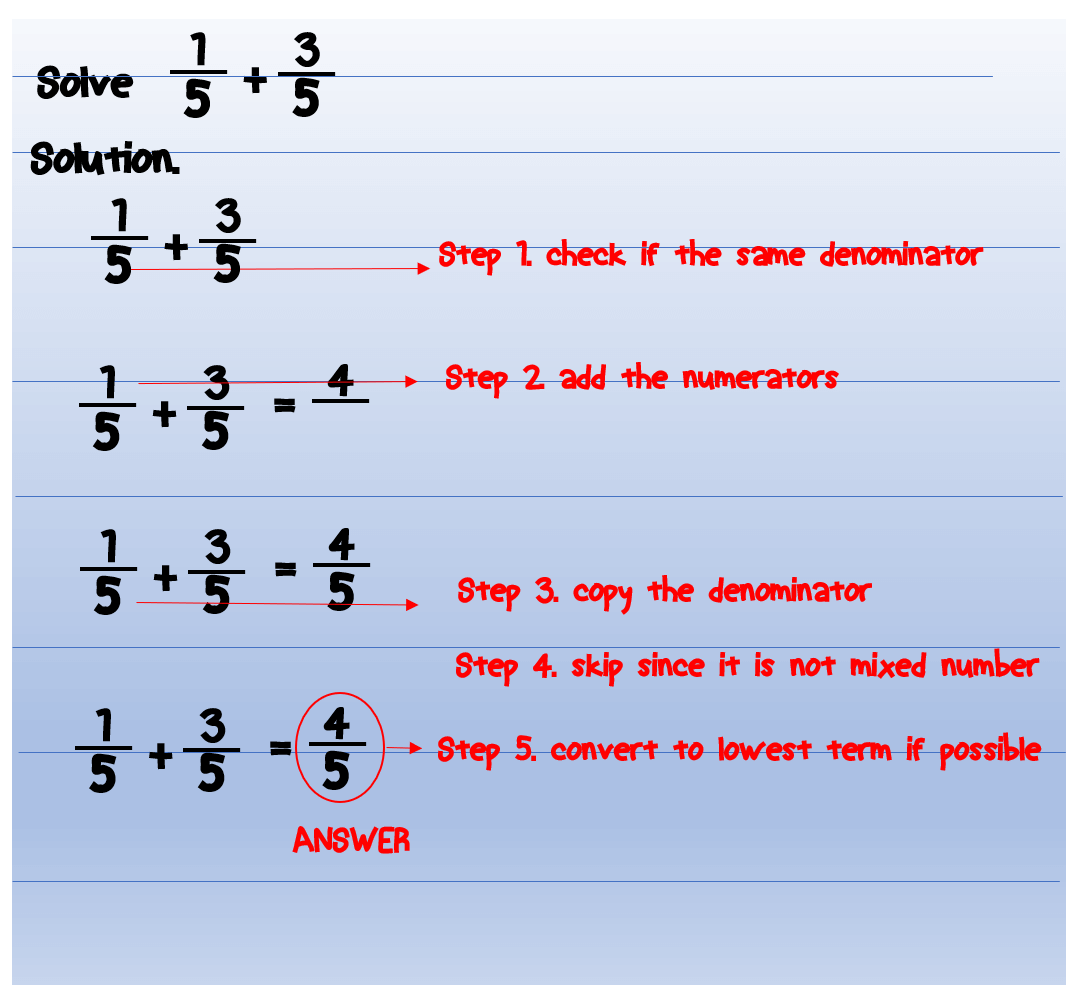Solve 1/5 +3/5.

Step 1 is to check the denominators. All the denominators are the same 5 thus we can proceed to step 2.

Step 2 is to add all the numerators. Let us add 1 and 3. This will give us 4.

Step 3 is to copy the denominator. Our denominator is 5.

Step 4 is to add whole numbers. Since we do not have mixed numbers, then we can skip this step.

Step 5 is to convert our answer into its lowest term. Since 4/5 is already the lowest term, then 4/5 is our final answer.

Example no.2 Let us try to add more proper fractions.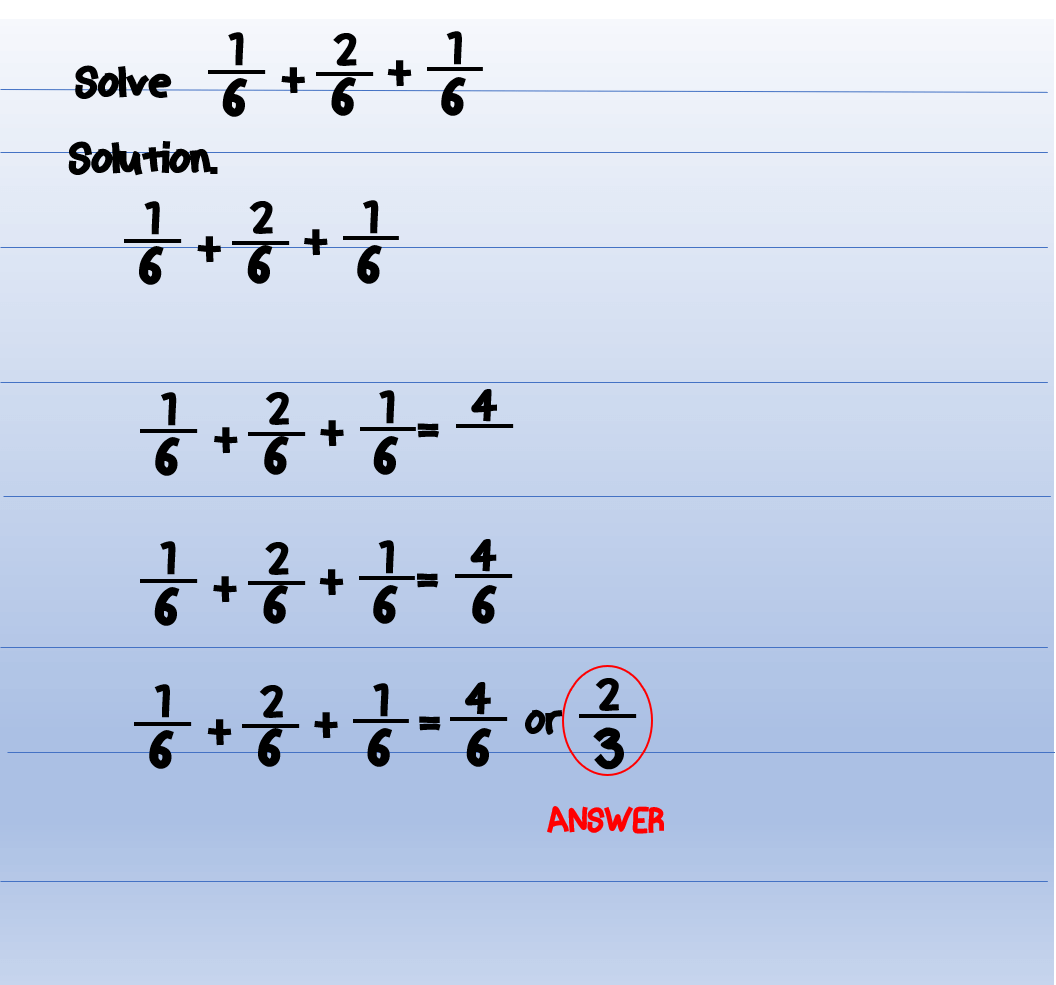Example no. 3 Let’s have another example.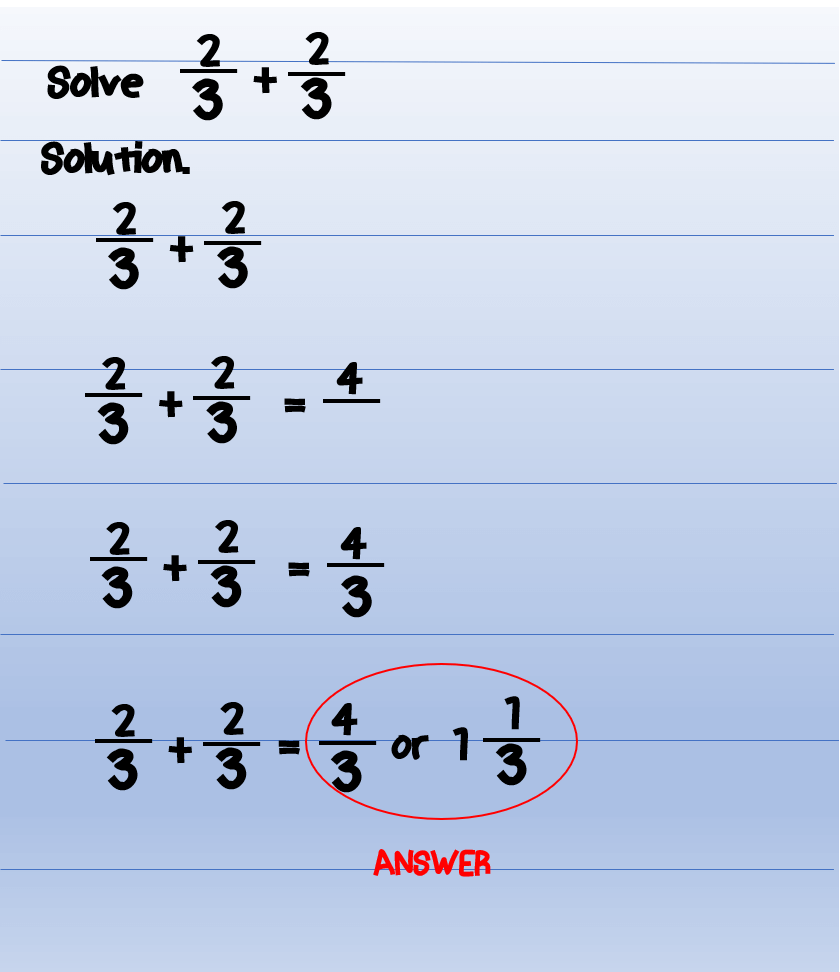Example no. 4 The same procedure will be applied when adding improper fractions with the same denominator.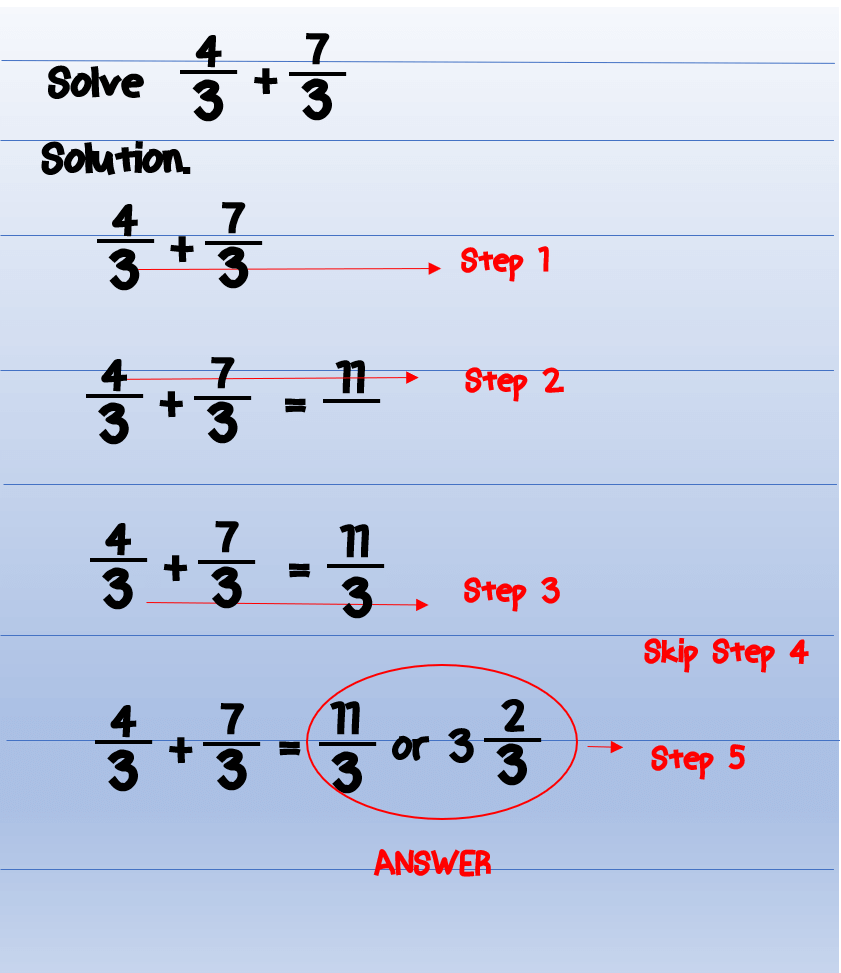Example no. 5 Let’s take a look at another example of adding similar fractions. This time, we are going to add a proper fraction and an improper fraction. We will follow the same steps.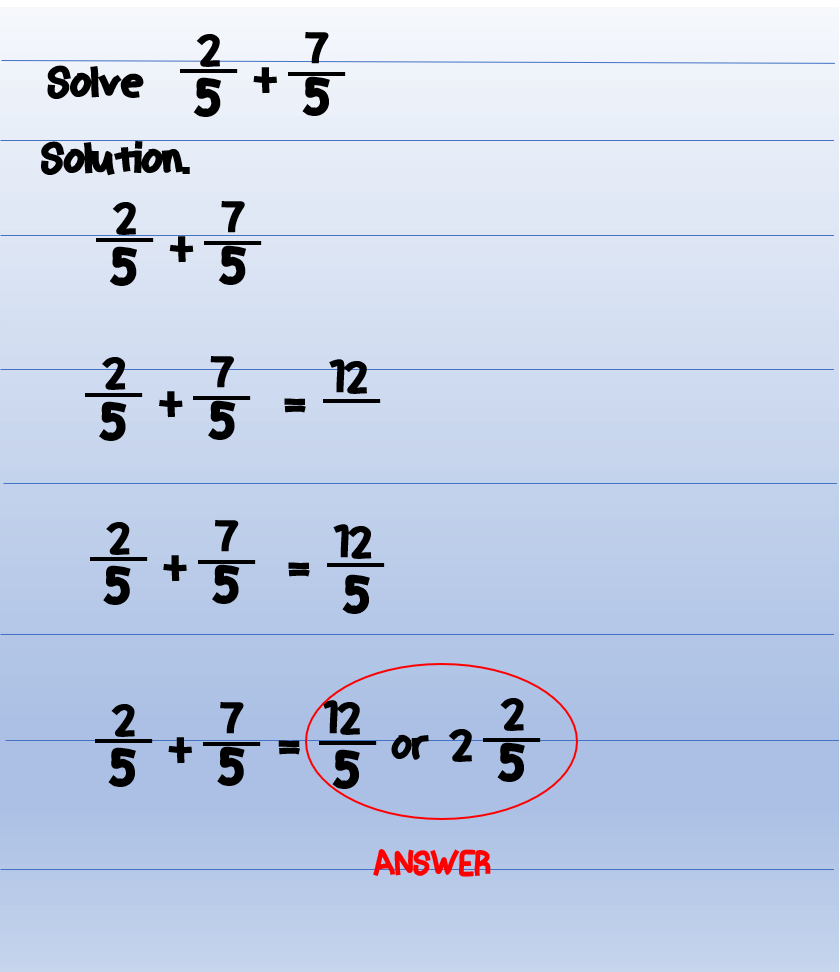Example no. 6 This time, let us add mixed numbers. The easiest way to add mixed numbers is to add them according to our procedure. Add the proper fractions and then the whole numbers. See the following example.Example no. 7 However, there are some problems in which after adding proper fractions, the result will be an improper fraction. Mixed numbers must have only a whole number and a proper fraction. If the partner of your whole number is an improper fraction, then you need to convert the improper fraction into mixed numbers and combine the whole numbers. See the example below.Note: 7/5 is not a proper fraction. A mixed number should have only a proper fraction and a whole number. Therefore, we need to convert it into a mixed number.

In this problem, it is better to convert the mixed numbers into an improper fraction and do the addition according to our procedure to avoid confusion. Let us solve example no.7 again.For me, this is way more easy than the previous one. But, whatever technique you are comfortable to use with, it will still give you the correct answer.

Example no. 8 We can also add a mixed number and a proper fraction. The procedure is the same as example no.6.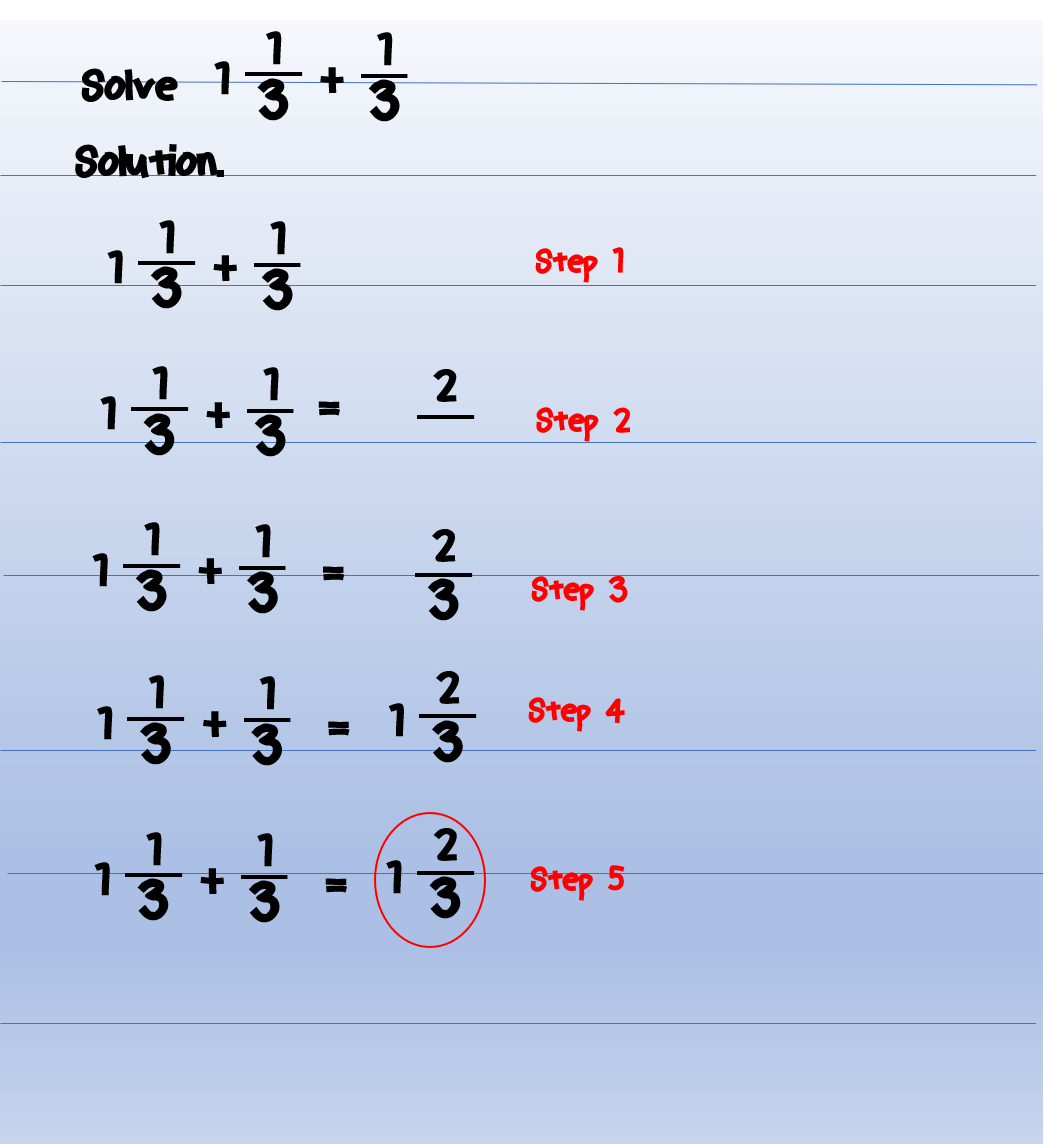If the result after adding the proper fractions is more than one, then follow the procedure in example no.7. Let us see another example.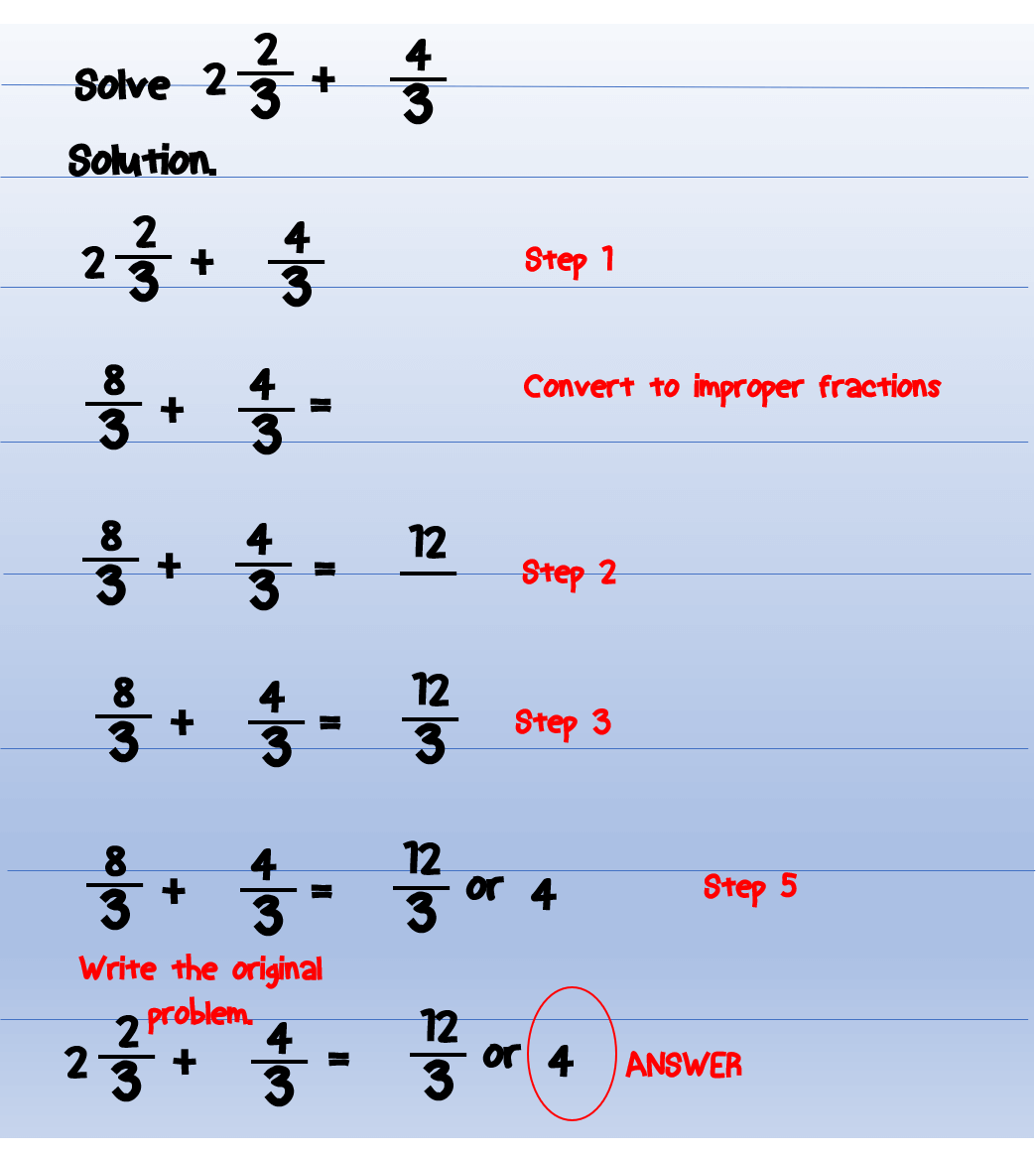Example no.10 Now, let us add similar fractions of different types.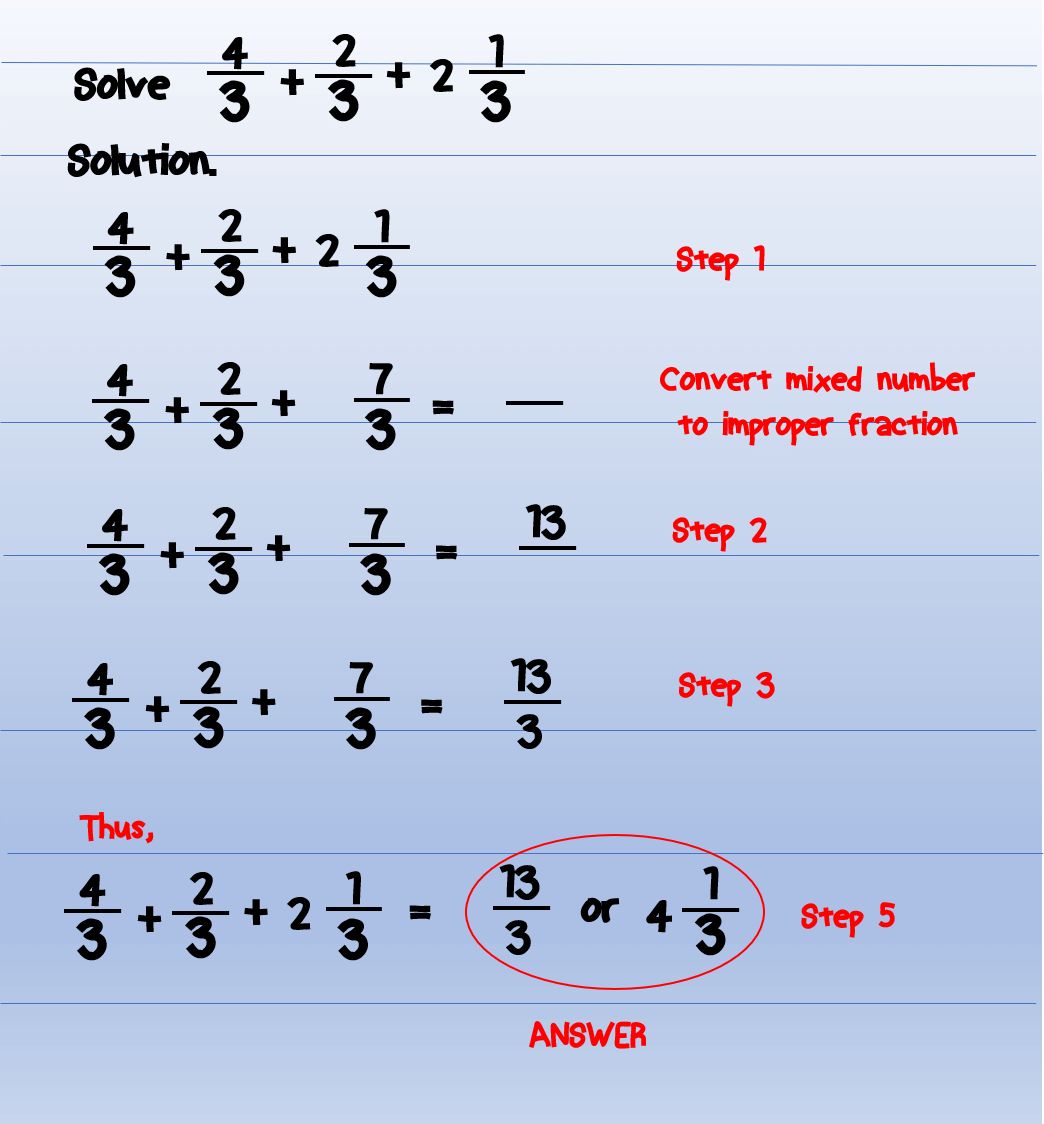### How to Subtract Similar Fractions

Dealing with the addition of similar fraction is quite simple. You just have to ensure that the denominators are the same so that you can proceed to add the numerators.

Subtracting similar fractions have the same procedure with adding similar fractions. The only difference is that you will be dealing with subtraction.

Let’s start.

### Subtracting Similar Fractions Examples:

Example no.11 Subtracting proper fractions with the same denominators.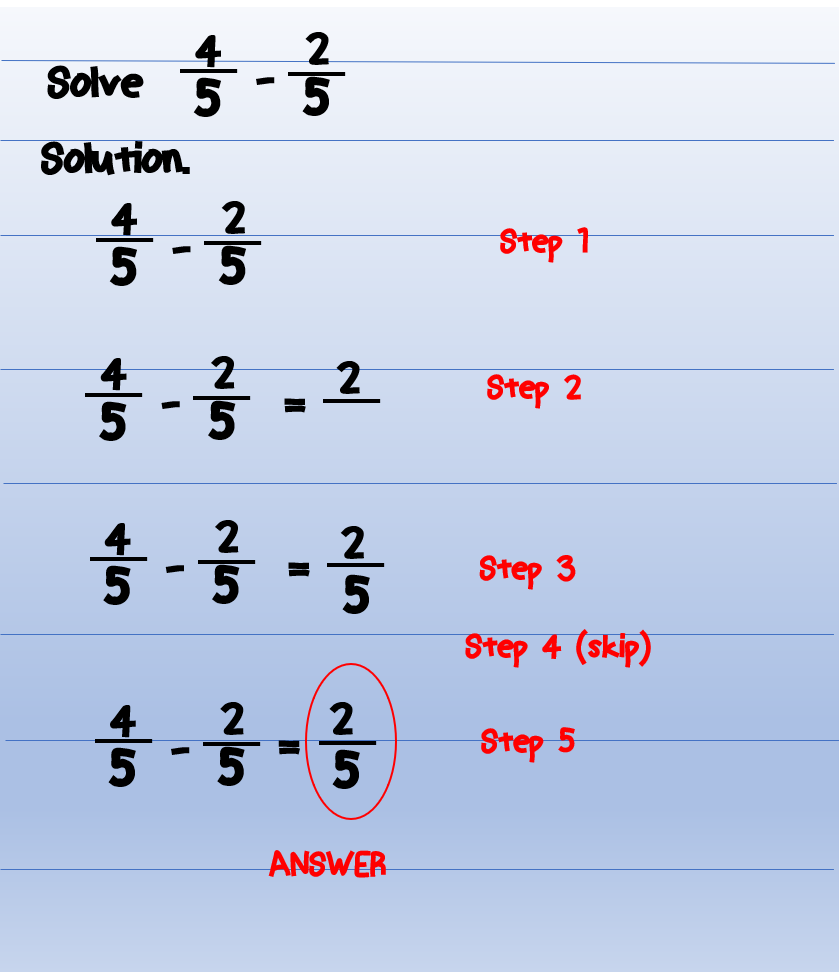Observe that we just follow the same procedure as the addition of fractions with the same denominators.

Example no.12 We can have also negative fractions as our results. Negative fractions are fractions which are less than zero.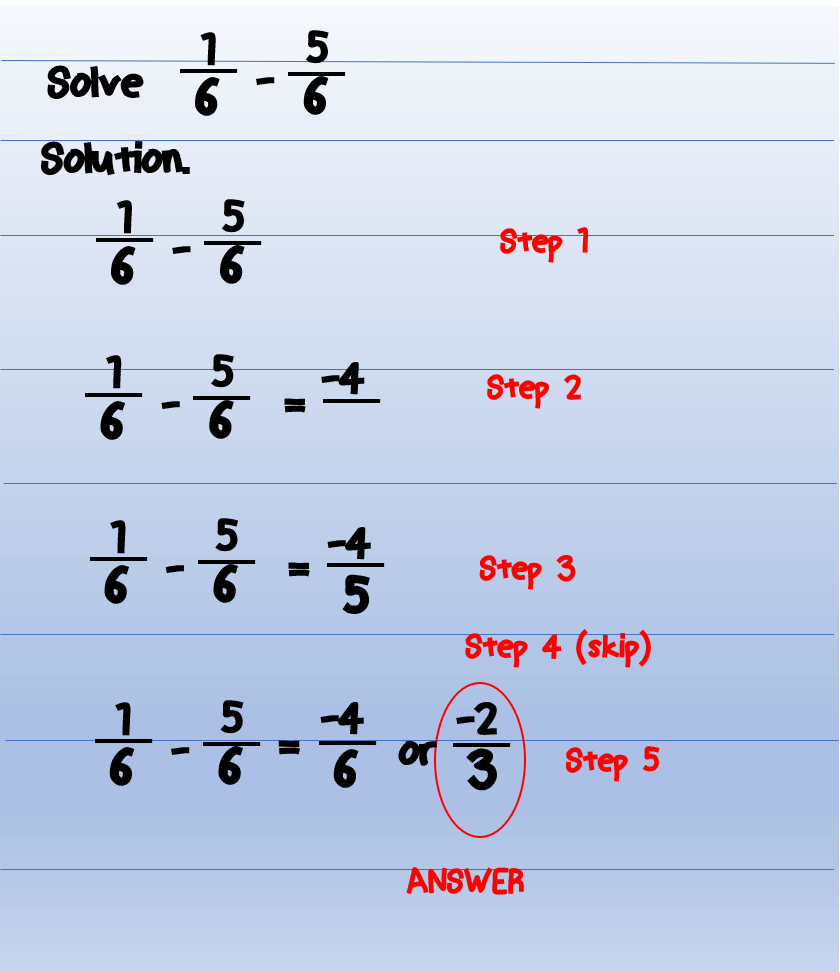Example no.13 Subtracting improper fractions with the same denominators.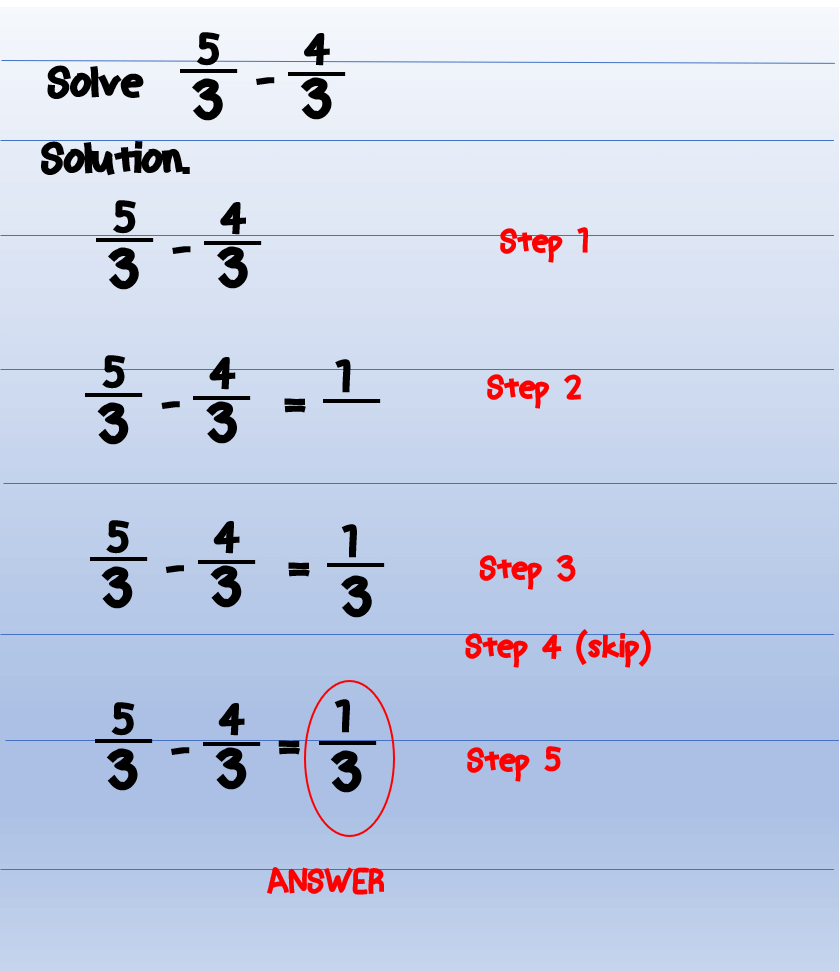Example no.14 In this example, we will subtract mixed numbers of similar fractions. It will be easy if we will convert mixed numbers into improper fractions and proceed with our procedure.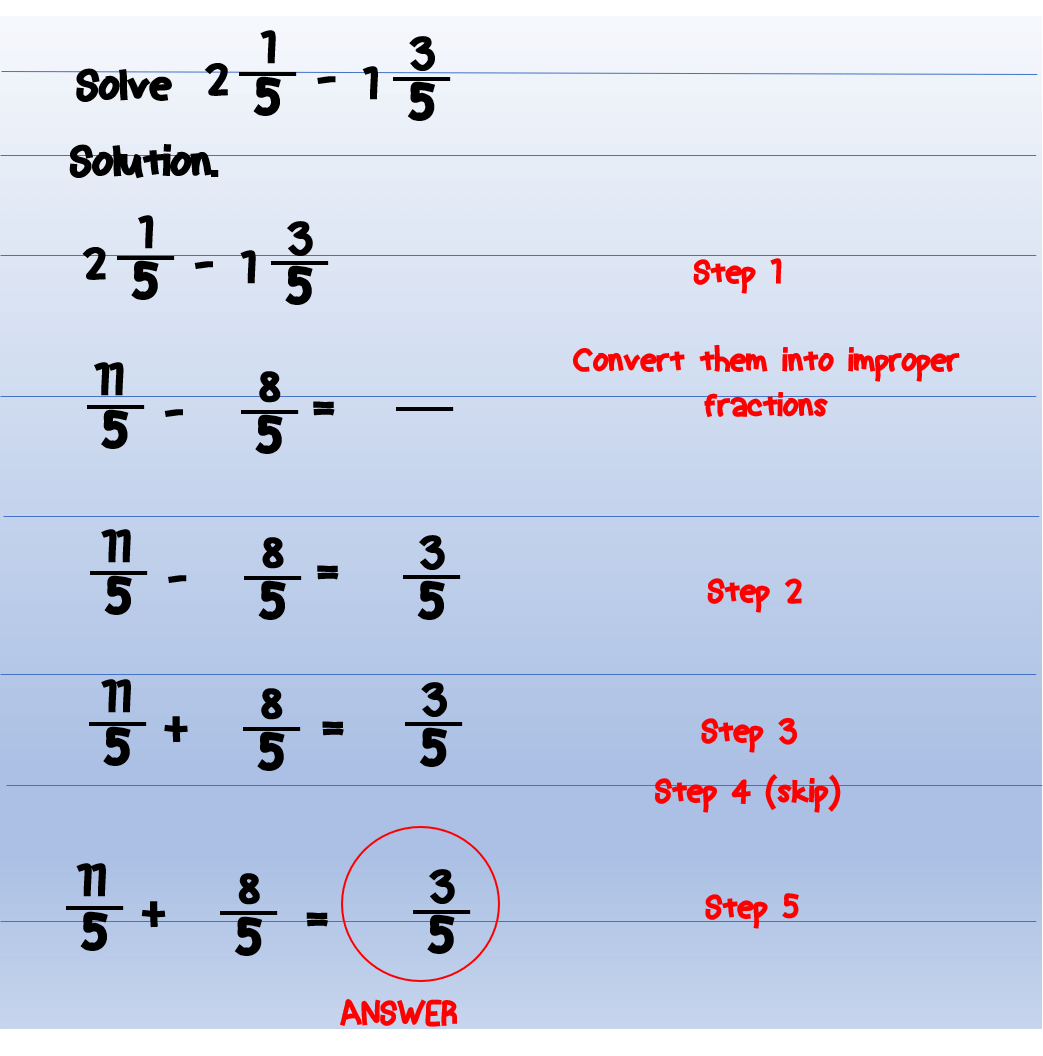Example no.15 More examples subtracting similar fractions.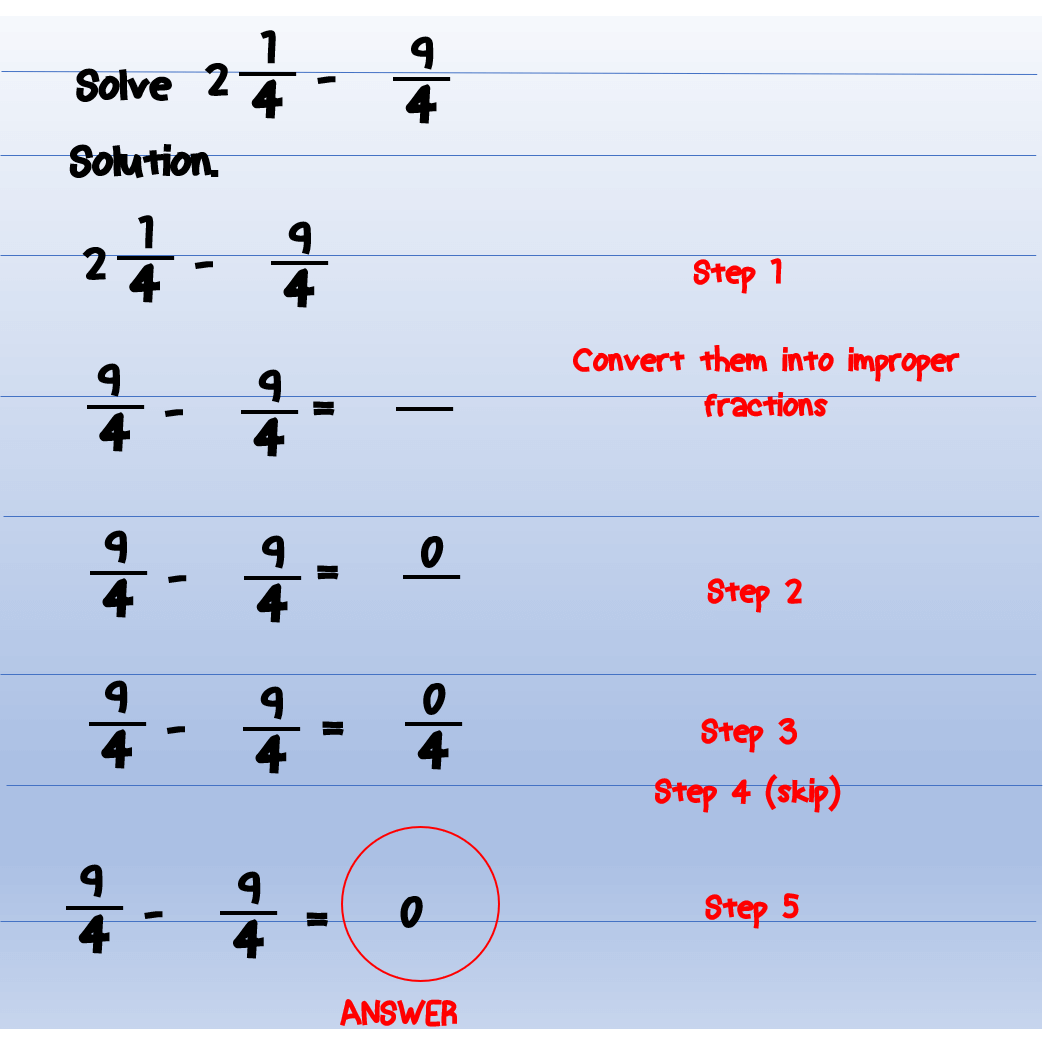### Summary of Adding and Subtracting Fractions

1. To add or subtract similar fractions, just add or subtract all the numerators and copy the denominators. Then, convert the answer to lowest term or mixed number.

2. For adding or subtracting mixed numbers with similar denominators, first, convert mixed numbers into improper fractions. Then, add or subtract the numerators and just copy the denominators. Convert your answer to lowest term or mixed number.

### Related Topics

If you have some questions or problems related to the above topic, please feel free to drop those in the comments section below. You can also send your concerns to our support team at support@math-lover.com.

The math-lover.com is happy to have you here reading this post.

1.FractionCalc says:

Adding and Subtracting numbers is time consuming and can exhaust too much energy on thinking what is the right answer. If practicing how to add and subtract numbers, it is better to do it manually but when you are in an actual situation that you are doing arithmetic operation, it is better to use calculator. Using calculator is not bad and it is a good strategy to save your time and physical strength plus you can be sure that you got the correct answer.

2.Russel John Regner says:

Masarap basahin Ito dahil marami Kang natutuhan sa subtract at sa iba ang math.

3.Giulianna Mharee L. Cristobal says:

I want to learn math.

4.ju says:

1.Faisal says:
5.nj moahludi says: